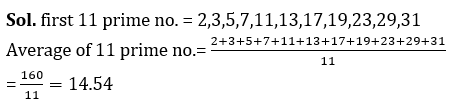Mathematics is an equally important section for CTET, MPTET, KVS & DSSSB Exams and has even more abundant importance in some other exams conducted by central or state govt.

To let you make the most of Mathematics section, we are providing important facts related to the Average. At least 1-2 questions are asked from this topic in most of the teaching exams. We wish you all the best of luck to come over the fear of the Mathematics section.

Mathematics Study Notes For All Teaching Exams

## Average

The average is nothing but the sum of all observations divided by the number of observations. This is also known as Arithmetic mean of the given observations or average value or mean value.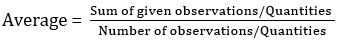Example: In an office, there are 10 female employees at the age of 35 years, 15 male employees at the age of 42 years. What is the average age of all employees?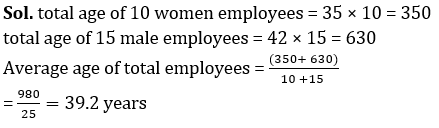Important terms:

Average of a given term is always lies in range at given data=Lowest Quantity ≤ Average ≤ Greatest quantity

• If the quantities of given data are equal then the average will also be the same as quantities. i.e. Average = Greatest / Lowest quantity

Value of all quantities = Average

• If ‘0’ is one of the quantities of a given data, then that ‘0’ will also be included while calculating average.

Average Speed : If a person covers a certain distance at a speed of A km/h and again cover the same distance at a speed of B km/h, then the average speed during the whole journey will be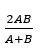If distance ‘A’ is covered with speed ‘a’ , distance ‘B’ is covered with speed ‘b’ and distance ‘C’ is covered with speed ‘c’, then for the whole journey: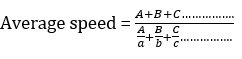Example: A boy covers 18 km at the speed of 9 km/hr, 32 km at 8 km /hr. and 60 km at 5 km/hr. Find the average speed of the total distance.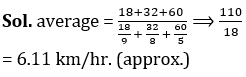Mathematics Quiz For CTET Exam : Attempt Daily Quizzes

AN EASY METHOD TO CALCULATE AVERAGES

The calculation of averages can be simplified greatly by taking some arbitrary number (P) as a starting point, take the deviations (differences) of the given items (Qi) from this arbitrary number, find the average of all these deviations (Qi – P) and algebraically add it to the arbitrary number (P) to give the correct average of the given items. If there are ‘n’ items and they are denoted by Q­1, Q2, Q3 ………… Qn, then the average of these ‘n’ items is given by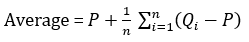The extent to which this method will simplify the calculation will depend on the selection of the arbitrary value P. It should be selected in such a way that the positive and negative deviations cancel out each other to the extent possible. Then the final figure left for division will be relatively small making the division easier.

Example: The average batting score of a player in his 100 innings is 70. His highest score is 122 runs more than that of his lowest score. If both these scores are removed his average batting score for 98 innings becomes 68. The highest score of the player is

Sol. Total runs of 100 innings = 70

Total runs in 98 innings excluding the highest and lowest runs = 7000 – 6664 = 336

x + x – 122 = 336

2x  = 458

x = 229

WEIGHTED AVERAGE

When two groups of Parts or objects are combined together, then we can talk of the average of the entire group. However, if we know only the average of the two groups individually, we cannot find out the average of the combined group of objects.

For example, there are two sections X and Y of a class where the average height of section X is 150 cm and that of section Y is 160 cm. On the basis of this information alone, we cannot find the average of the entire class (of the two sections).

How To Score 25+ Marks In Mathematics For CTET Exam?

the average height of the entire class is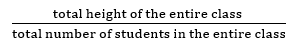Since we do not have any information regarding the number of students in the two sections, we cannot find the average of the entire class. Now, suppose that we are given that there are 60 students in the section X and 40 students in section Y, then we can calculate the average height of the entire class which, in this case will be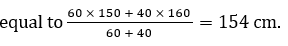This average height 154 cm of the entire class is called “weighted average” of the class.

Helping Points:

Sum of arithmetic progression whose first term is “a” last term is [a + (n – 1)d].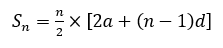Sum of geometric progression whose first term is [a], last term is

and common ratio is (r)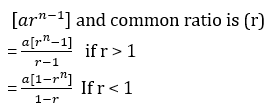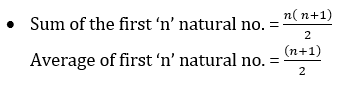Practice More Mathematics  Quiz Here

Example: If the average of 6 even consecutive numbers is 27. Find the largest number

Sol. let the smallest even number = x

x+x+2+x+4+x+6+x+8+x+10= 27×6

6x+30= 162

6x= 132

x= 22

Largest number = x+10

= 22+10= 32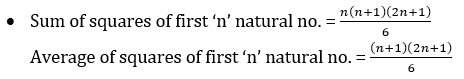Example: Find the average of square of first 15 natural numbers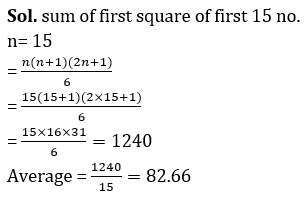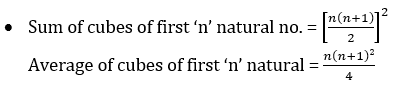How to Overcome Exam Fever, Especially When You Fear Maths

Example: Find the average of cubes of natural numbers till 64.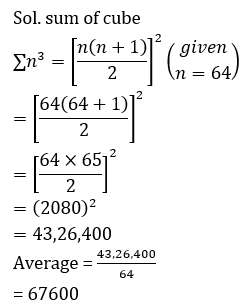• Sum of first ‘n’ natural odd numbers = n²

Average of first ‘n’ natural odd numbers = n

Example: Average of first 7 odd multiples of 3 is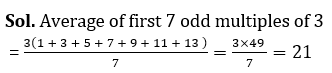• Sum of first n natural Even numbers = n(n +1)

Average of first ‘n’ natural Even numbers = (n +1)

Example: Find the average of the first 11 prime numbers